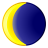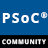# What is the difference between I2S Data bits vs Word select periodLevel 3
Level 3Hi,

I am bit confused about the word select period. The I2S module data sheet says about bit resolution as "This parameter determines the number of data bits configured for each sample. If the Static Bit resolution is selected, this value can be set between 8 and 32." For example, I decided to use 16 bit for each sample, so my data bits should be 16 bits. Now what is the word select period?

From data sheet, "This parameter determines the period of a complete sample of both left and right channels. This value can be set to 16, 32, 48, or 64 (default)". So in my case it is again 16,  I believe I2S send send data as <I2S Left Sample><I2S Right Sample><I2S left sample>....

So why there two parameters to set?

1 Solution

# Re: What is the difference between I2S Data bits vs Word select periodEmployee
EmployeeTh word select period tells how many bits will be in the I2S frame (left and right).

The bit resolution tells how many bits the sample has.

The word select period has to be higher or equal to the bit resolution. If the word select period is higher than the bit resolution, the remaining bits are set to ZERO or ONEs.

# Re: What is the difference between I2S Data bits vs Word select periodEmployee
EmployeeTh word select period tells how many bits will be in the I2S frame (left and right).

The bit resolution tells how many bits the sample has.

The word select period has to be higher or equal to the bit resolution. If the word select period is higher than the bit resolution, the remaining bits are set to ZERO or ONEs.